# How would you describe the Structure of an Atom

## How would you describe the Structure of an Atom

After the discovery of electron and proton, the scientists started thinking of arranging these particles in an atom. J.J. Thomson was the first scientist to propose a model for the structure of atom. Mainly there are three model preposed.

1. Thomson’s model of an atom
2. Rutherford model of an atom
3. Bohr’s model of an atom

1. Thomson’s model of an atom
Thomson was the first to propose a detailed model of the atom. He proposed that an atom consists of a uniform sphere of positive electricity in which the electrons are distributed more or less uniformly. The negative and the positive charge are equal in magnitude. Thus, the atom as a whole is electrically neutral. This model of atom is known as the “Plum pudding model”.2. Rutherford model of an atom
3. Bohr’s model of an atom

## The Electronic Structure of an Atom

1. Electrons are arranged around the nucleus in the shells of an atom.
2. The shells of an atom are numbered 1,2, 3 and so on, starting from the one closest to the nucleus.
3. Each shell can occupy a certain number of electrons, as shown in Figure below. For atoms with proton numbers of 1 to 20, two electrons can occupy the first shell, eight electrons in the second shell and eight electrons in the third shell. Any additional electrons will be placed in the fourth shell.
Figure: Maximum number of electrons that can occupy each shell for elements with the proton numbers of 1 to 20.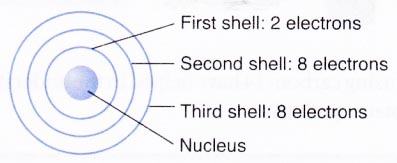## Finding the electron arrangement

To write the electron arrangement of an atom, follow these steps:
Step 1 Find out the proton number of the atom. (From Periodic Table)
Step 2 Find out the number of electrons of the atom.
For a neutral atom, the number of electrons is the same as the number of protons.
Step 3 Arrange the electrons in the shells. Electrons occupy the shells closest to the nucleus first. Electrons only start occupying a new shell when the previous one has been occupied.

## Finding the electron arrangement Example Problems with Solutions

1. What is the electron arrangement of a chlorine atom?
Solution:
The proton number of chlorine is 17.
The number of electrons in a chlorine atom is 17.
The 17 electrons are arranged as two in the first shell, eight in the second shell and the remaining seven in the third shell.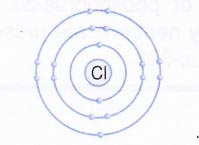The electron arrangement of the chlorine atom L . can be written as 2.8.7.

2. What is the electron arrangement of a potassium atom?
Solution:
The proton number of potassium is 19.
Hence, the number of electrons in a potassium atom is 19.
The 19 electrons are arranged as two in the first shell, eight in the second shell, eight in the third shell and the remaining one in the fourth shell.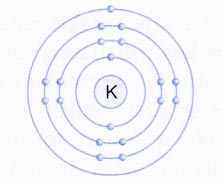The electron arrangement can be written as 2.8.8.1.
Note:

• Electrons can be represented as dots or crosses.
• Always check to make sure that the electrons add up to the right number – in this case, 19.

## Valence electrons

1. Valence electrons are electrons found in the outermost occupied shell of an atom.
2. The outermost occupied shell is the shell which is furthest from the nucleus.
3. The number of valence electrons in an atom can be determined from its electron arrangement.
4. For example, the electron arrangement of a chlorine atom is 2.8.7. There are 7 electrons in the outermost occupied shell of the chlorine atom. Therefore, the number of valence electrons in a chlorine atom is 7.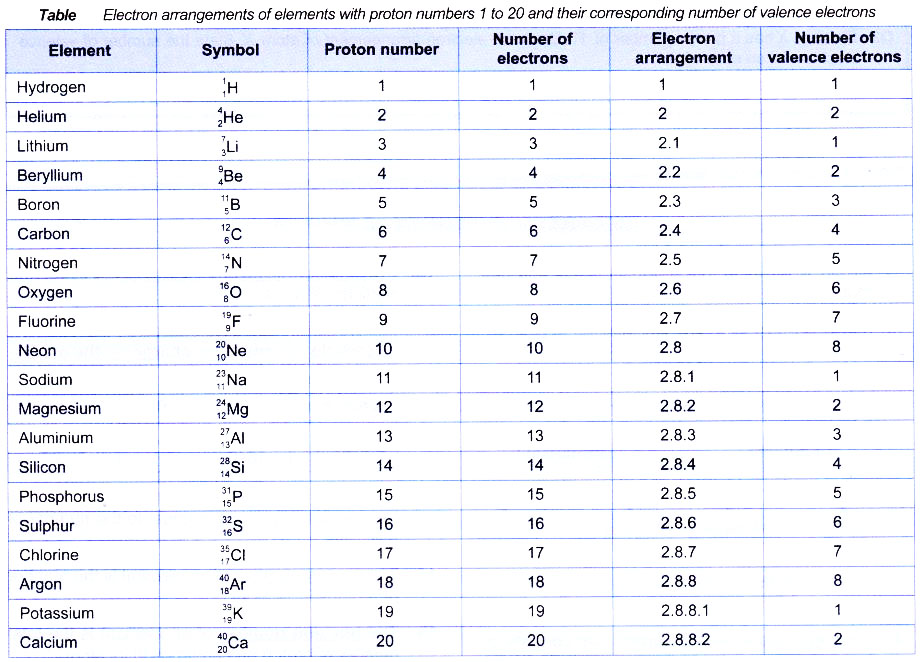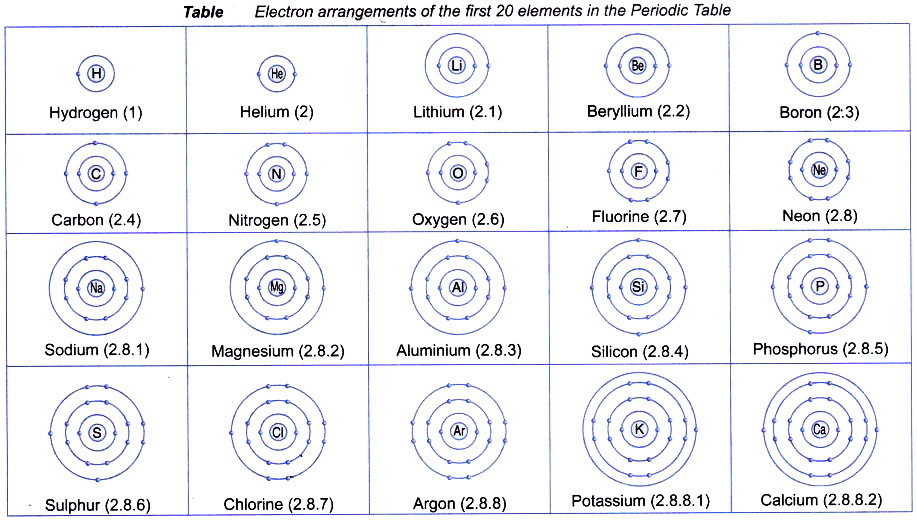## Atomic number

The number of protons present in the nucleus of an atom of an element is known as its atomic number.
Example: An atom of carbon has 6 protons so, the atomic number of carbon is 6. The atomic number is denoted by Z. Now positive charge on the nucleus is due to the presence of protons in it and each proton carries one unit of positive charge. Therefore, the atomic number of an element is equal to number of positive charges carried by the nucleus of an atom of the element.
Atomic number of an element (Z)
= Number of protons (p)
= Number of positive charges carried by the nucleus of the atom
or = Number of electrons (e)

## Mass number

The sum of the number of protons and neutrons in an atom of the element.
Mass number (A) = No. of protons (p) + No. of neutrons (n)
Example: Helium atom has 2 protons, 2 neutrons and 2 electron. Its mass number is equal to 2 + 2 = 4.
Example: Oxygen has 8 protons, 8 neutrons and 8 electrons. its mass number is 16.
In some cases, particularly heavier elements, the number of neutrons is more than the number of protons.
Example: Mercury has atomic number equal to 80. So, it has 80 protons and 80 electrons. But the mass number of mercury is 200. Therefore, the number of neutrons in mercury is 200 – 80 = 120.
Generally, an atom is represented by its symbol for the element. Atomic number is written on the lower side of the symbol and the mass number is written on the upper side.A = Mass number
Z = Atomic number
X = Symbol of element
Example: Indicates that lithium has atomic number equal to 3 and mass number equal to 7.

## Relationship between mass number and atomic number

Mass number = No. of protons + No. of neutrons
Mass number = Atomic number + No. of neutrons
Example 1: The atomic nucleus of an element has mass number 23 and number of neutrons 12. What is the atomic number of the element ?
Solution: We know that
Mass No. = No. of protons + No. of neutrons
23 = No. of protons + 12
∴ No. of protons = 23 – 12 = 11
Now, Atomic No. = No. of protons = 11.

Example 2: Calculate the number of :
(i)  electrons                  (ii) protons
(iii) neutrons and            (iv) nucleons
Solution: Mass No. = 39
Atomic No. = 19
(i)   We know that
atomic No. = No. of protons= No. of electrons
No. of electrons = 19
(ii)  No. of protons = 19
(iii) Mass No. = No. of neutrons + No. of electrons
No. of neutrons = mass number – No. of protons
= 39 – 19 = 20
(iv) Nucleons = No. of protons + No. of neutrons
= 19 + 20 = 39